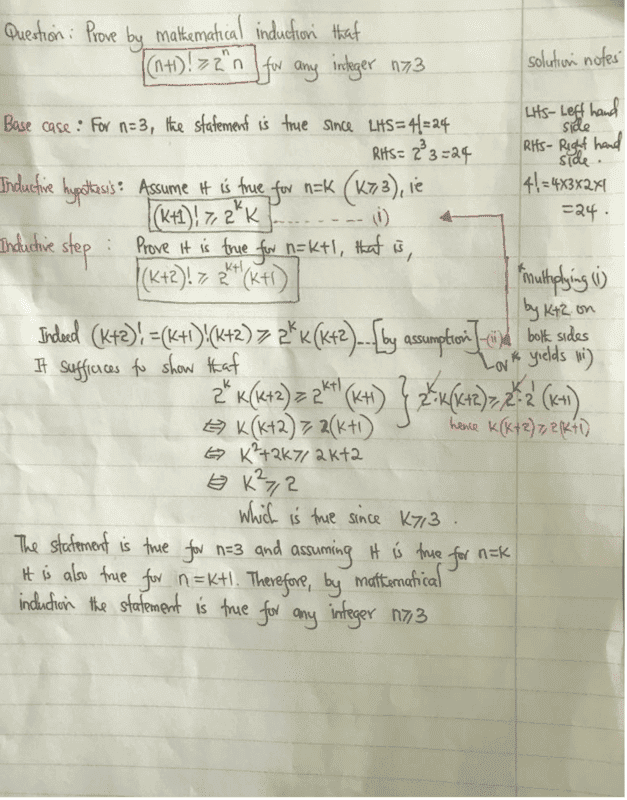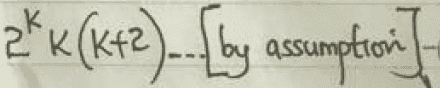# Proof by induction problem

• chwala

#### chwala

Gold Member
Homework Statement
prove that,

##(n+1)!≥ 2^n. n## when ## n≥3##
Relevant Equations
mathematics inductionthis is a solution posted by my colleague, i have a problem in understanding how he got to conclude on equation (ii)is this not supposed to be ##(k+2)!≥ 2^{k+1} (k+1)##
##(k+2)(k+1)!≥ 2^{k+1} (k+1)## ?...

Assume that ##(k+1)! \geq 2^k k##. Then notice that ##(k+2)! = (k+2)(k+1)!##, and since ##(k+1)! \geq 2^k k## by assumption, you have ##(k+2)! = (k+2)(k+1)! \geq (k+2)2^k k##.

You're trying to show that ##(k+2)!## is greater than ##2^{k+1}(k+1)## given that it holds for the case below, so you can't assume what's meant to be proven!

Homework Statement:: prove that,

##(n+1)!≥ 2^n. n## when ## n≥3##
Relevant Equations:: mathematics induction

View attachment 269193
this is a solution posted by my colleague, i have a problem in understanding how he got to conclude on equation (ii)
View attachment 269194
is this not supposed to be ##(k+2)!≥ 2^{k+1} (k+1)##
##(k+2)(k+1)!≥ 2^{k+1} (k+1)## ?...
The inductive assumption is that ##(k+1)!≥ 2^{k} (k)##, hence:
$$(k+2)! = (k+2)(k+1)! \ge (k+2)2^k (k)$$

•chwala
aaaaaaah i have seen it...

•etotheipi
There must be a neater way to do this. That proof you attached is quite laboured.

There must be a neater way to do this. That proof you attached is quite laboured.

you mind showing a neater way now that i am conversant with how the solution was found...

You just need to state that ##k(k+2) > 2(k+1)## for all ##k \geq 3##. Then it follows that$$(k+2)! \geq k(k+2)2^k > 2(k+1) 2^k = (k+1)2^{k+1}$$

•chwala and PeroK
For the inductive step, all that is required is:
$$(k+2)! = (k+2)(k+1)! \ge (k+2)2^k(k) > (k+1)2^{k+1} \ \ \ (\text{as} \ k + 2 > k+1 \ \text{and} \ k > 2)$$

•chwala and etotheipi